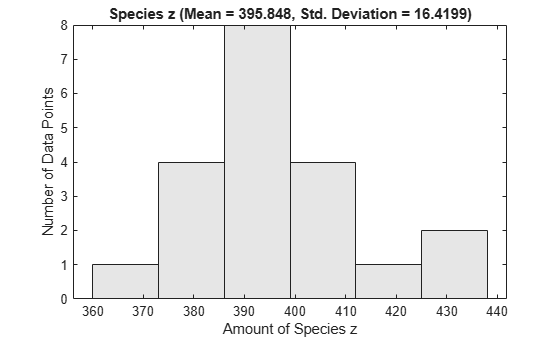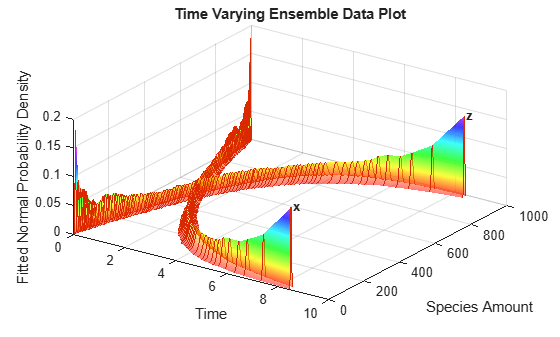# sbioensemblestats

Get statistics from ensemble run data

## Syntax

```[t,m] = sbioensemblestats(simDataObj) [t,m,v] = sbioensemblestats(simDataObj) [t,m,v,n] = sbioensemblestats(simDataObj) [t,m,v,n] = sbioensemblestats(simDataObj,names) [t,m,v,n] = sbioensemblestats(simDataObj,names,interpolation) ```

## Arguments

 `t` Column vector of time points `m` Matrix of mean values from the ensemble data. The number of rows in `m` is the length of the time vector `t` and the number of columns is equal to the number of species. `simDataObj` A cell array of SimData objects, where each SimData object holds data for a separate simulation run. All elements of `simDataObj` must contain data for the same states in the same model. When the time vectors of the elements of `simDataObj` are not identical, `simDataObj` is first resampled onto a common time vector (see `interpolation` below). `v` Matrix of variance obtained from the ensemble data. `v` has the same dimensions as `m`. `n` Cell array of character vectors for the quantity names whose mean and variance are returned in `m` and `v`, respectively. The number of elements in `n` is the same as the number of columns of `m` and `v`. The order of names in `n` corresponds to the order of columns of `m` and `v`. `names` Character vector, string, string vector, string array, or cell array of character vectors. `names` may include qualified names such as `'CompartmentName.SpeciesName'` or `'ReactionName.ParameterName'` to resolve ambiguities. If you specify empty `{}` or empty string array (`string.empty`) for `names`, `sbioensemblestats` returns statistics on all time courses contained in `simDataObj`. `interpolation` Character vector or string denoting the interpolation method to use for resampling of the data onto a common time vector with the smallest simulation stop time. See `resample` for a list of interpolation methods. Default is `'linear'`.

## Description

`[t,m] = sbioensemblestats(simDataObj)` computes the time-dependent ensemble mean `m` of the ensemble data `simDataObj`. If the time vectors of the ensemble data are not identical, by default, the function uses the `'linear'` interpolation method to resample the data onto the common time vector. See `resample` for a list of interpolation methods.

`[t,m,v] = sbioensemblestats(simDataObj)` also returns the variance `v` for the ensemble run data `simDataObj`.

`[t,m,v,n] = sbioensemblestats(simDataObj)` also returns the names of quantities `n` corresponding to the mean `m` and variance `v` columns. Each column of `m` or `v` describes the ensemble mean or variance of a quantity (or state) as a function of time.

`[t,m,v,n] = sbioensemblestats(simDataObj,names)` computes statistics only for the quantities specified by `names`.

`[t,m,v,n] = sbioensemblestats(simDataObj,names,interpolation)` uses the interpolation method `interpolation` to resample the simulation data to have a consistent time vector. If the time vectors of the ensemble data are not identical and if you do not specify any interpolation method, the function uses the `'linear'` interpolation method by default.

## Examples

collapse all

`sbioloadproject("radiodecay.sbproj");`

Change the solver of the active configuration set to be `ssa`. Also, adjust the `LogDecimation` property on the `SolverOptions` property of the configuration set.

```cs = getconfigset(m1,"active"); cs.SolverType = "ssa"; so = cs.SolverOptions; so.LogDecimation = 10;```

Perform an ensemble of 20 runs with no interpolation.

```ensembleData = sbioensemblerun(m1,20); sbioensembleplot(ensembleData, 'z', 1.0);```Create a 2-D distribution plot of the species z at time = 1.0.

`sbioensembleplot(ensembleData,"z",1.0); `Get ensemble statistics for all species using the default interpolation method.

`[T,M,V] = sbioensemblestats(ensembleData);`

Get ensemble statistics for a specific species using the default interpolation scheme.

`[T2,M2,V2] = sbioensemblestats(ensembleData,"z");`

Create a 3-D shaded plot of the species x and z.

`sbioensembleplot(ensembleData,["x","z"]);`## Version History

Introduced in R2006a